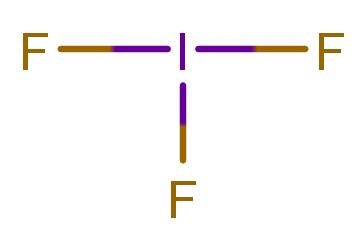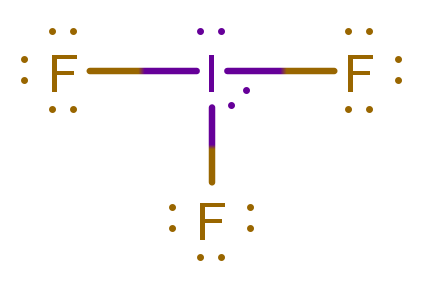# Problem: Predict the molecular geometries of the following species, respectively: IF3, TeF4, XeF51+. A. trigonal pyramidal, square planar, linear B. trigonal pyramidal, tetrahedral, see-saw C. trigonal planar, tetrahedral, square pyramidal D. T-shaped, see-saw, square pyramidal E. trigonal planar, see-saw, square planar

###### FREE Expert Solution

The possible molecular geometries are:For IF3:

Step 1: Iodine (EN = 2.66) is less electronegative than Fluorine (EN = 3.98) iodine is the central atom.

Step 2: The total number of valence electrons present in IF3 is:

Group             Valence Electrons

I             7A                   1 × 7 e7 e

F            7A                   3 × 7 e21 e

Total:  28 valence e

Step 3: I will go in the center while F atoms are terminal atoms (Recall that when halogens are not the central atom, they only form 1 bond.)

Let’s first connect each atom with single bonds.A bond corresponds to 2 electrons shared between two atoms so we currently have 3 × 2 e = 6 val. e, which means we’re still lacking 22 e. We can start adding the missing electrons to each atom while following the octet rule

The complete Lewis structure is:I has 10 e(exemption to the octet rule), and F: 8 e each

Step 4: The shape of a molecule is based on its molecular geometry: when determining molecular geometry, atoms and lone pairs are treated differently

95% (69 ratings)###### Problem Details

Predict the molecular geometries of the following species, respectively: IF3, TeF4, XeF51+.

A. trigonal pyramidal, square planar, linear

B. trigonal pyramidal, tetrahedral, see-saw

C. trigonal planar, tetrahedral, square pyramidal

D. T-shaped, see-saw, square pyramidal

E. trigonal planar, see-saw, square planar